# Regular Expressions Regular Expressions Notation to specify a

• Slides: 32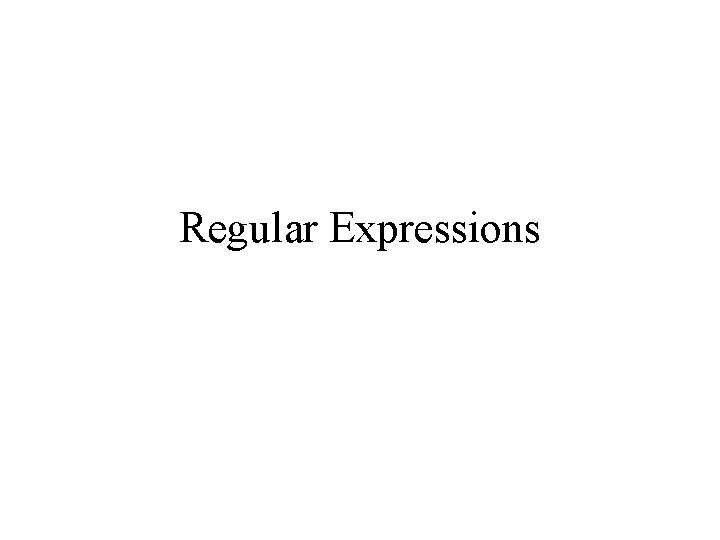Regular ExpressionsRegular Expressions • Notation to specify a language – Declarative – Sort of like a programming language. • Fundamental in some languages like perl and applications like grep or lex – Capable of describing the same thing as a NFA • The two are actually equivalent, so RE = NFA = DFA – We can define an algebra for regular expressionsAlgebra for Languages • Previously we discussed these operators: – Union – Concatenation – Kleene StarDefinition of a Regular Expression • R is a regular expression if it is: 1. 2. 3. 4. 5. 6. 7. a for some a in the alphabet , standing for the language {a} ε, standing for the language {ε} Ø, standing for the empty language R 1+R 2 where R 1 and R 2 are regular expressions, and + signifies union (sometimes | is used) R 1 R 2 where R 1 and R 2 are regular expressions and this signifies concatenation R* where R is a regular expression and signifies closure (R) where R is a regular expression, then a parenthesized R is also a regular expression This definition may seem circular, but 1 -3 form the basis Precedence: Parentheses have the highest precedence, followed by *, concatenation, and then union.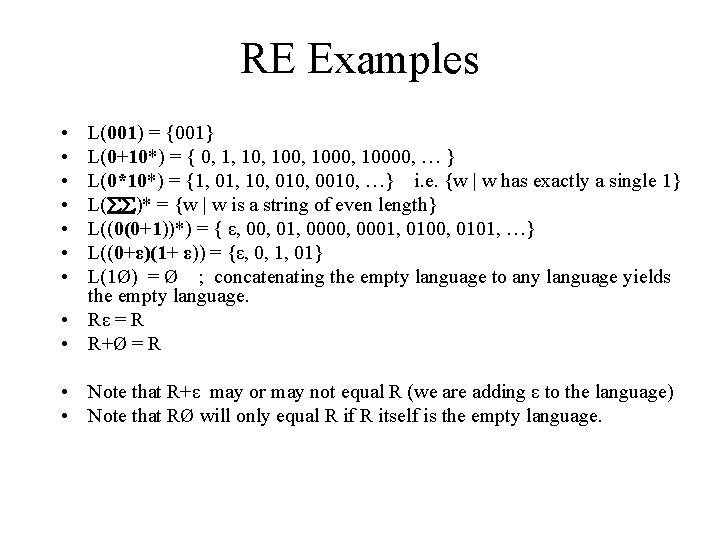RE Examples • • L(001) = {001} L(0+10*) = { 0, 1, 100, 10000, … } L(0*10*) = {1, 01, 10, 0010, …} i. e. {w | w has exactly a single 1} L( )* = {w | w is a string of even length} L((0(0+1))*) = { ε, 00, 01, 0000, 0001, 0100, 0101, …} L((0+ε)(1+ ε)) = {ε, 0, 1, 01} L(1Ø) = Ø ; concatenating the empty language to any language yields the empty language. • Rε = R • R+Ø = R • Note that R+ε may or may not equal R (we are adding ε to the language) • Note that RØ will only equal R if R itself is the empty language.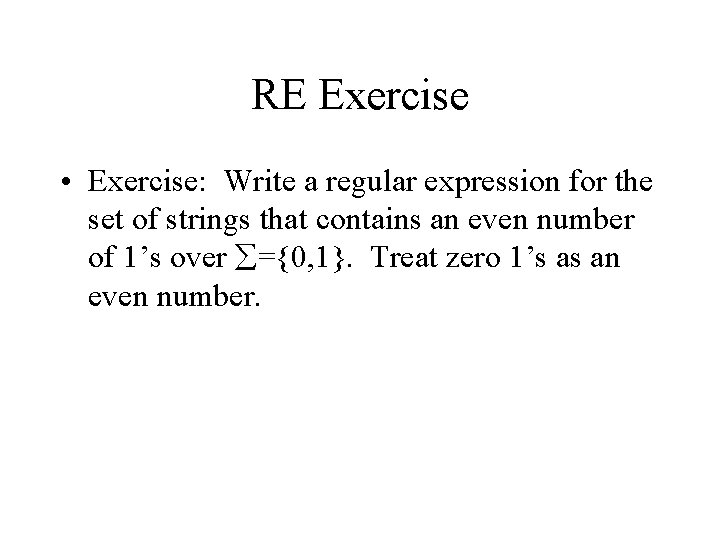RE Exercise • Exercise: Write a regular expression for the set of strings that contains an even number of 1’s over ={0, 1}. Treat zero 1’s as an even number.Equivalence of FA and RE • Finite Automata and Regular Expressions are equivalent. To show this: – Show we can express a DFA as an equivalent RE – Show we can express a RE as an ε-NFA. Since the ε-NFA can be converted to a DFA and the DFA to an NFA, then RE will be equivalent to all the automata we have described.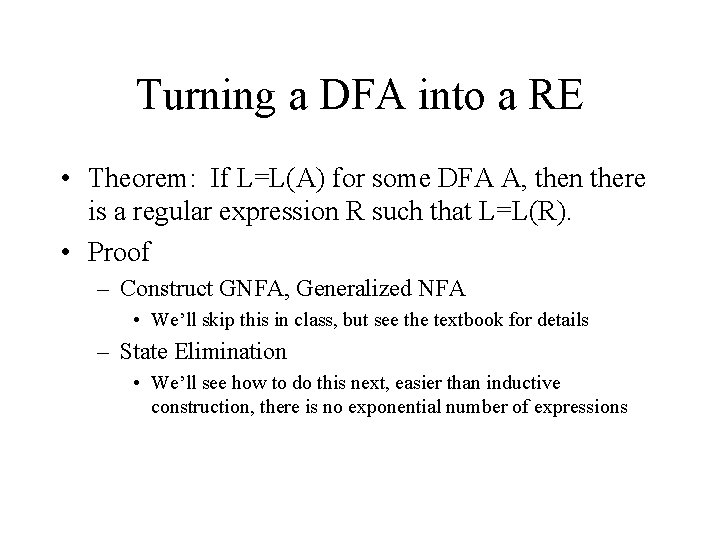Turning a DFA into a RE • Theorem: If L=L(A) for some DFA A, then there is a regular expression R such that L=L(R). • Proof – Construct GNFA, Generalized NFA • We’ll skip this in class, but see the textbook for details – State Elimination • We’ll see how to do this next, easier than inductive construction, there is no exponential number of expressions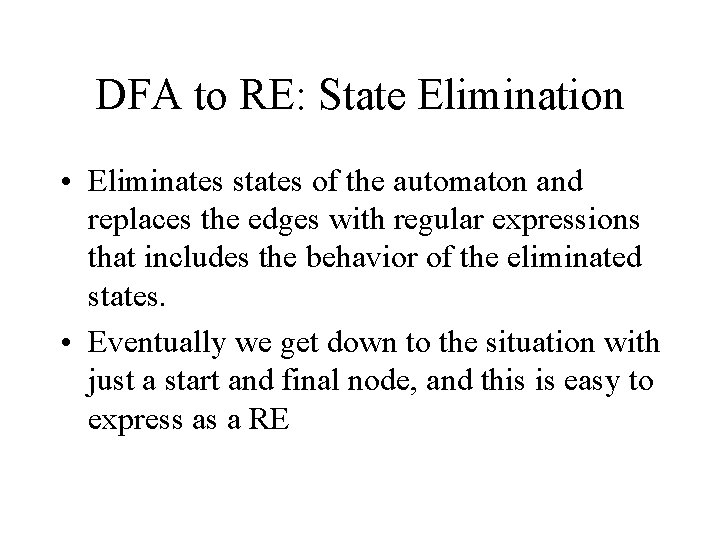DFA to RE: State Elimination • Eliminates states of the automaton and replaces the edges with regular expressions that includes the behavior of the eliminated states. • Eventually we get down to the situation with just a start and final node, and this is easy to express as a REState Elimination • • Consider the figure below, which shows a generic state s about to be eliminated. The labels on all edges are regular expressions. To remove s, we must make labels from each qi to p 1 up to pm that include the paths we could have made through s. Note: q and p may be the same state!DFA to RE via State Elimination (1) 1. Starting with intermediate states and then moving to accepting states, apply the state elimination process to produce an equivalent automaton with regular expression labels on the edges. • The result will be a one or two state automaton with a start state and accepting state.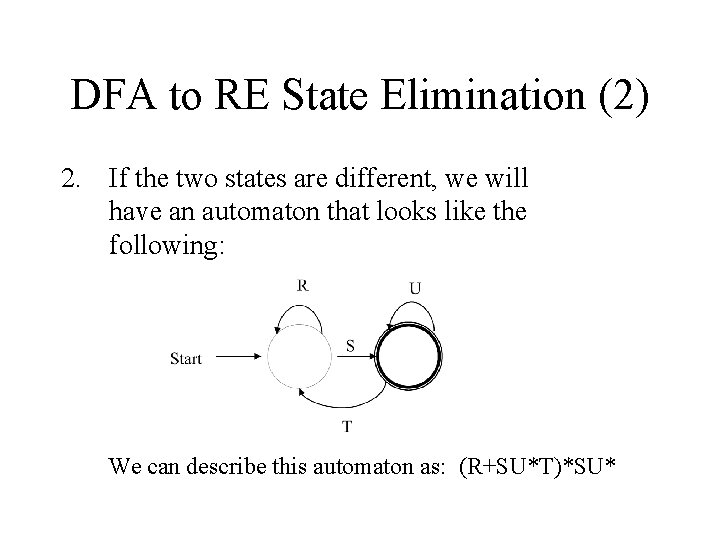DFA to RE State Elimination (2) 2. If the two states are different, we will have an automaton that looks like the following: We can describe this automaton as: (R+SU*T)*SU*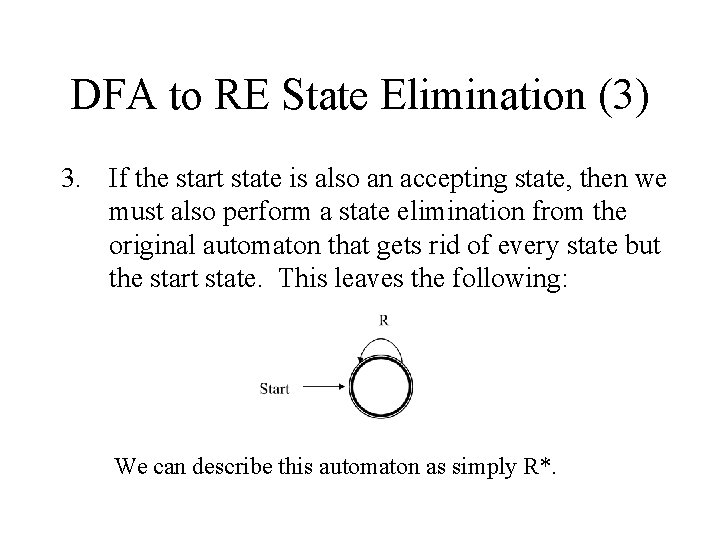DFA to RE State Elimination (3) 3. If the start state is also an accepting state, then we must also perform a state elimination from the original automaton that gets rid of every state but the start state. This leaves the following: We can describe this automaton as simply R*.DFA to RE State Elimination (4) 4. If there are n accepting states, we must repeat the above steps for each accepting states to get n different regular expressions, R 1, R 2, … Rn. For each repeat we turn any other accepting state to non-accepting. The desired regular expression for the automaton is then the union of each of the n regular expressions: R 1 R 2… RN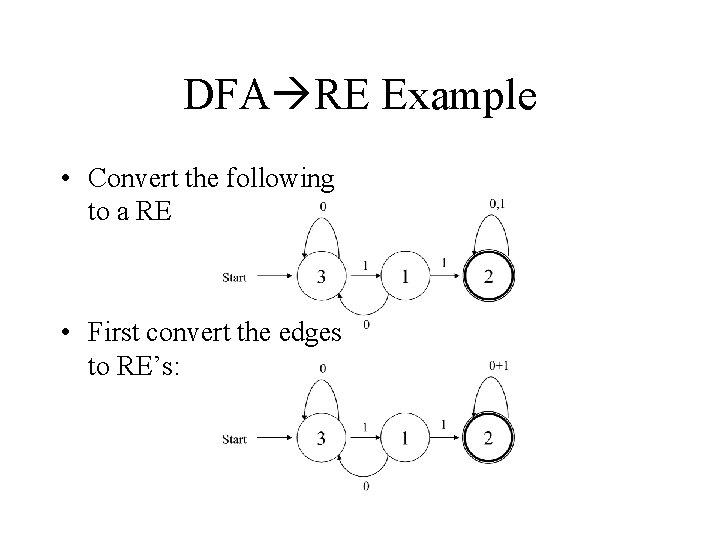DFA RE Example • Convert the following to a RE • First convert the edges to RE’s: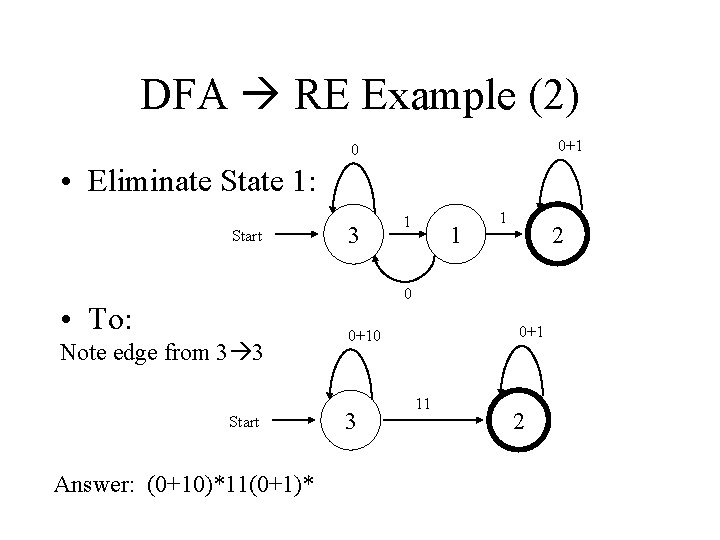DFA RE Example (2) 0+1 0 • Eliminate State 1: Start 3 1 1 1 2 0 • To: Note edge from 3 3 Start Answer: (0+10)*11(0+1)* 0+10 3 11 2Second Example • Automata that accepts even number of 1’s 0 0 Start 1 1 • Eliminate state 2: 1 1 3 1 0+10*1 0 Start 2 0 10*1 3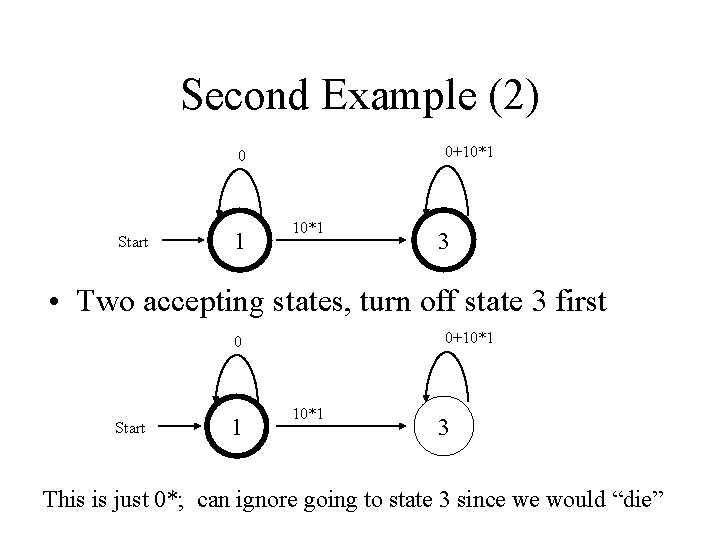Second Example (2) 0+10*1 0 Start 1 10*1 3 • Two accepting states, turn off state 3 first 0+10*1 0 Start 1 10*1 3 This is just 0*; can ignore going to state 3 since we would “die”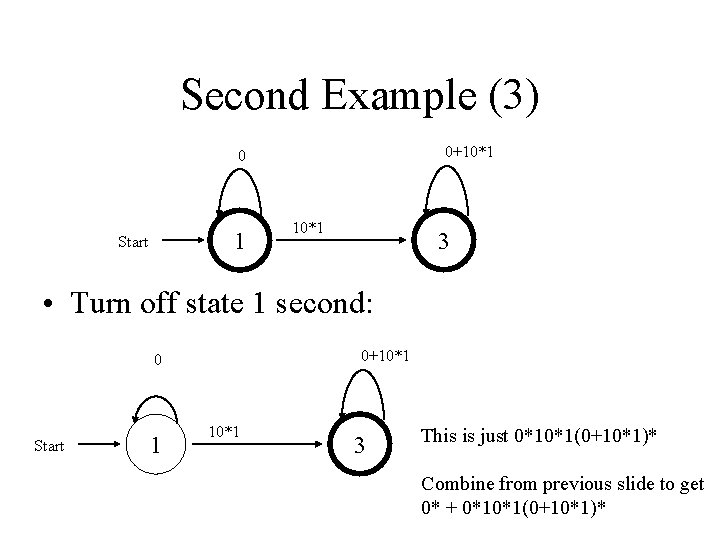Second Example (3) 0+10*1 0 1 Start 10*1 3 • Turn off state 1 second: 0+10*1 0 Start 1 10*1 3 This is just 0*10*1(0+10*1)* Combine from previous slide to get 0* + 0*10*1(0+10*1)*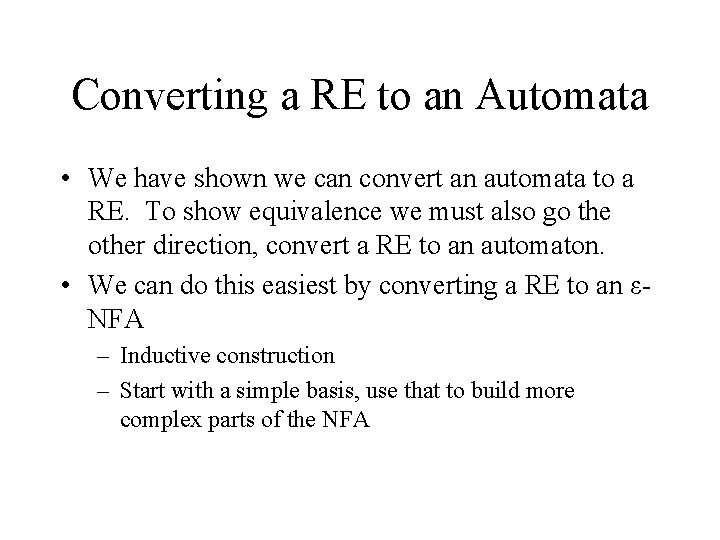Converting a RE to an Automata • We have shown we can convert an automata to a RE. To show equivalence we must also go the other direction, convert a RE to an automaton. • We can do this easiest by converting a RE to an εNFA – Inductive construction – Start with a simple basis, use that to build more complex parts of the NFA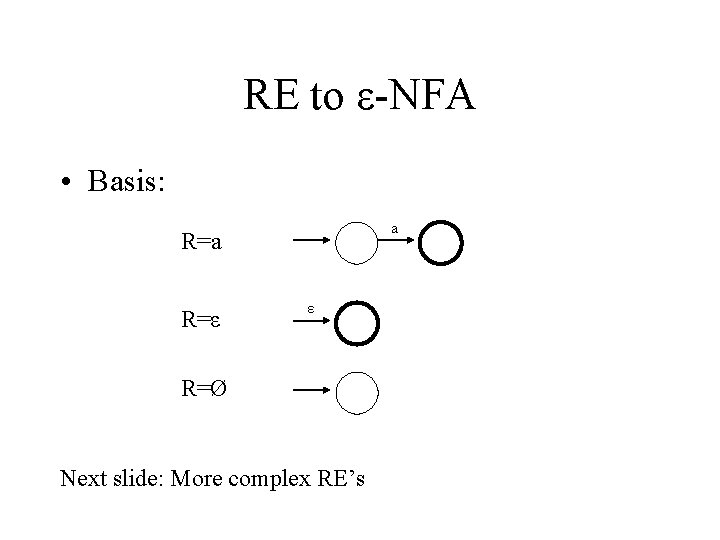RE to ε-NFA • Basis: a R=ε ε R=Ø Next slide: More complex RE’s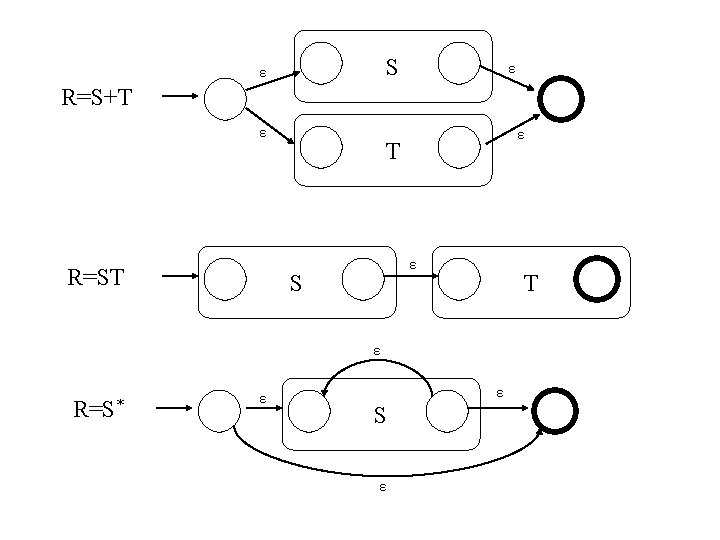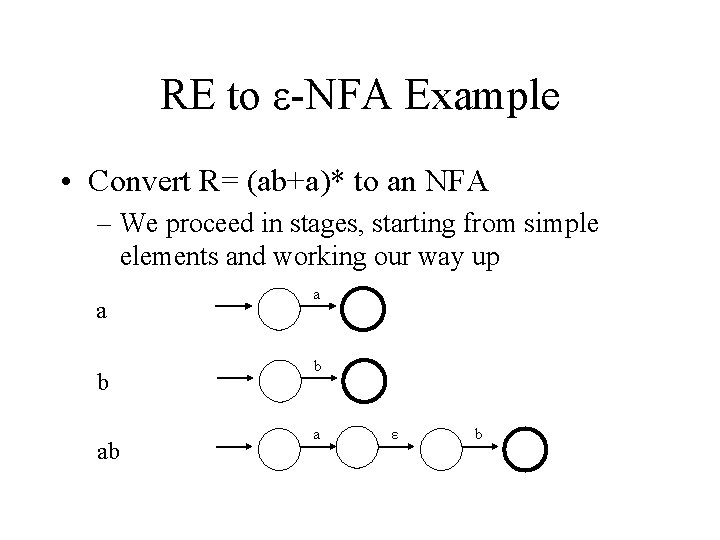RE to ε-NFA Example • Convert R= (ab+a)* to an NFA – We proceed in stages, starting from simple elements and working our way up a b ab a ε b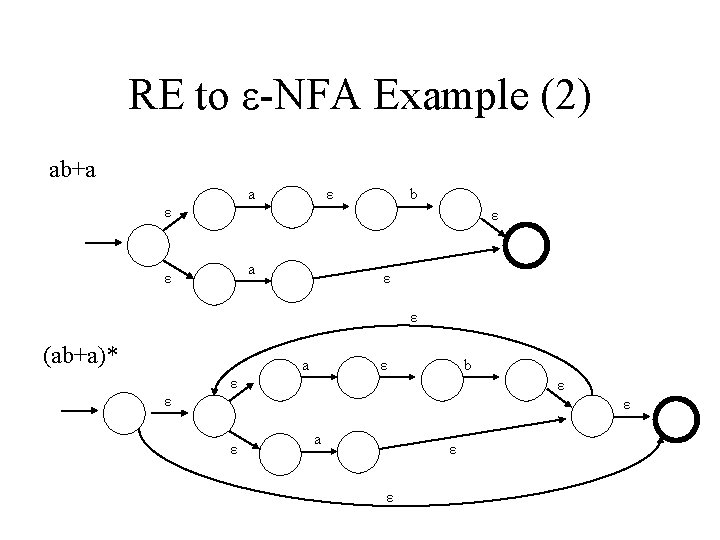RE to ε-NFA Example (2) ab+a a ε b ε ε a ε ε ε (ab+a)* a ε b ε ε ε a ε ε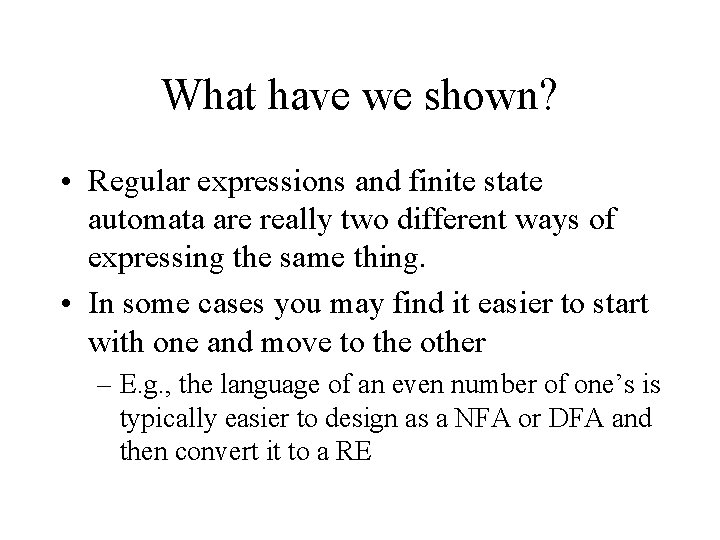What have we shown? • Regular expressions and finite state automata are really two different ways of expressing the same thing. • In some cases you may find it easier to start with one and move to the other – E. g. , the language of an even number of one’s is typically easier to design as a NFA or DFA and then convert it to a RE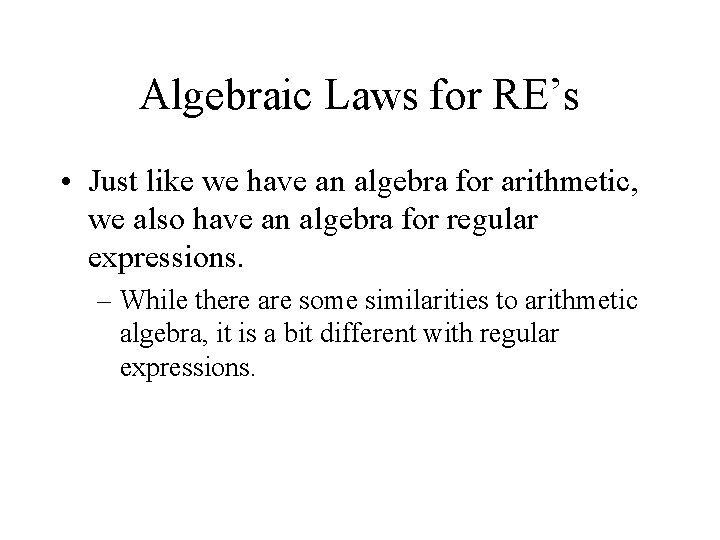Algebraic Laws for RE’s • Just like we have an algebra for arithmetic, we also have an algebra for regular expressions. – While there are some similarities to arithmetic algebra, it is a bit different with regular expressions.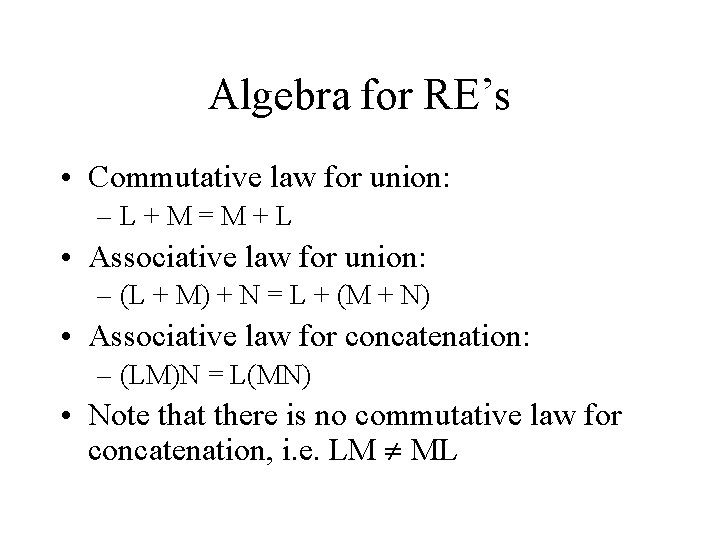Algebra for RE’s • Commutative law for union: –L+M=M+L • Associative law for union: – (L + M) + N = L + (M + N) • Associative law for concatenation: – (LM)N = L(MN) • Note that there is no commutative law for concatenation, i. e. LM MLAlgebra for RE’s (2) • The identity for union is: – L+Ø=Ø+L=L • The identity for concatenation is: – Lε = εL = L • The annihilator for concatenation is: – ØL = LØ = Ø • Left distributive law: – L(M + N) = LM + LN • Right distributive law: – (M + N)L = LM + LN • Idempotent law: – L+L=L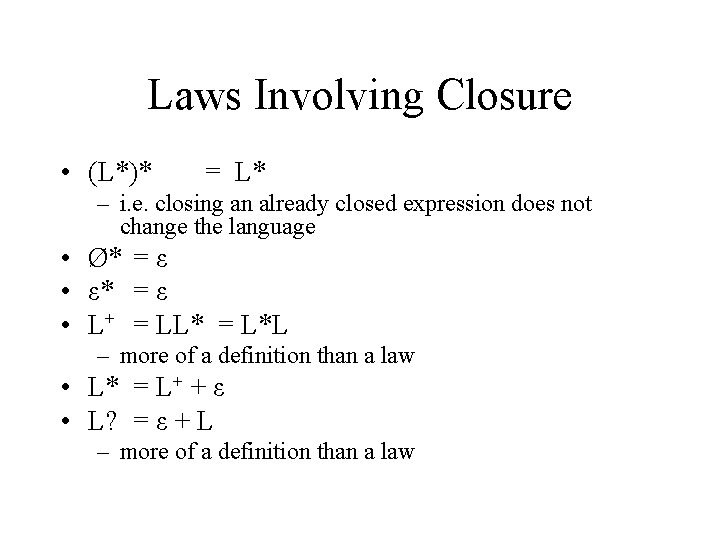Laws Involving Closure • (L*)* = L* – i. e. closing an already closed expression does not change the language • Ø* = ε • ε* = ε • L+ = LL* = L*L – more of a definition than a law • L* = L+ + ε • L? = ε + L – more of a definition than a law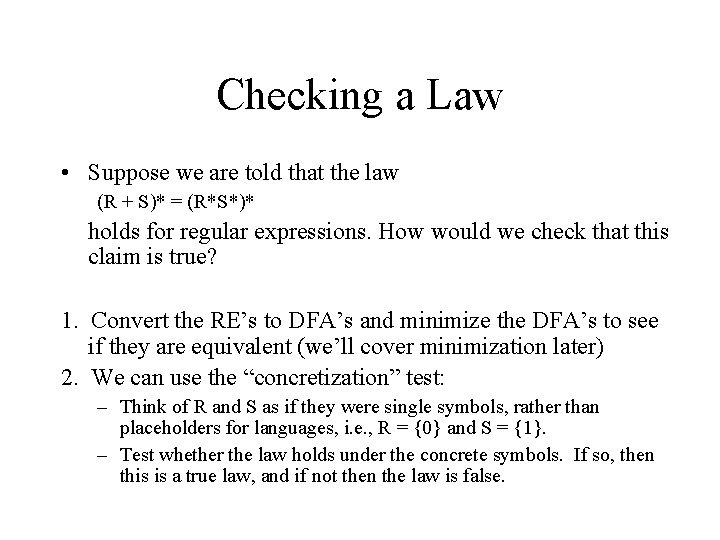Checking a Law • Suppose we are told that the law (R + S)* = (R*S*)* holds for regular expressions. How would we check that this claim is true? 1. Convert the RE’s to DFA’s and minimize the DFA’s to see if they are equivalent (we’ll cover minimization later) 2. We can use the “concretization” test: – Think of R and S as if they were single symbols, rather than placeholders for languages, i. e. , R = {0} and S = {1}. – Test whether the law holds under the concrete symbols. If so, then this is a true law, and if not then the law is false.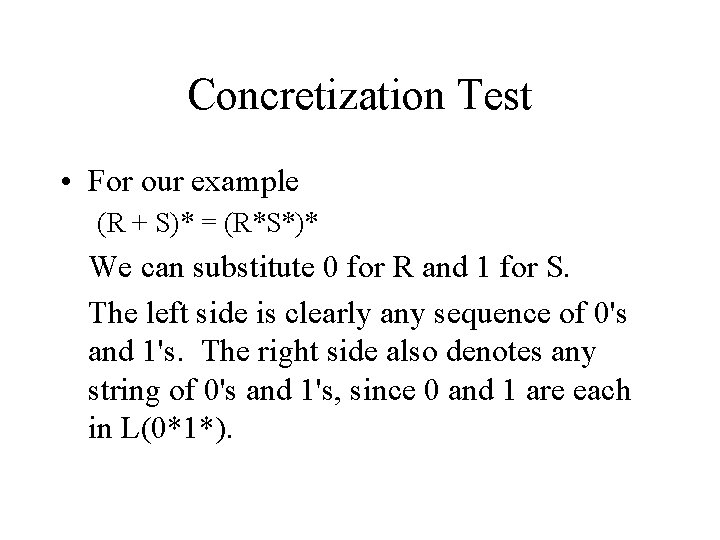Concretization Test • For our example (R + S)* = (R*S*)* We can substitute 0 for R and 1 for S. The left side is clearly any sequence of 0's and 1's. The right side also denotes any string of 0's and 1's, since 0 and 1 are each in L(0*1*).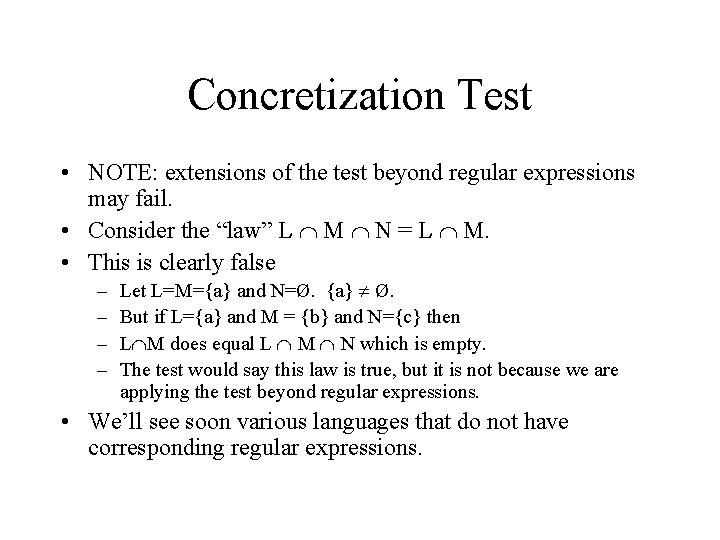Concretization Test • NOTE: extensions of the test beyond regular expressions may fail. • Consider the “law” L M N = L M. • This is clearly false – – Let L=M={a} and N=Ø. {a} Ø. But if L={a} and M = {b} and N={c} then L M does equal L M N which is empty. The test would say this law is true, but it is not because we are applying the test beyond regular expressions. • We’ll see soon various languages that do not have corresponding regular expressions.